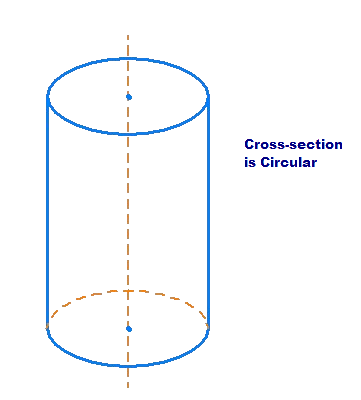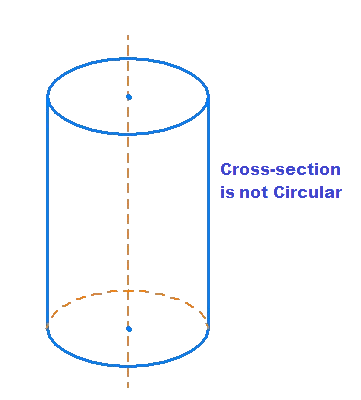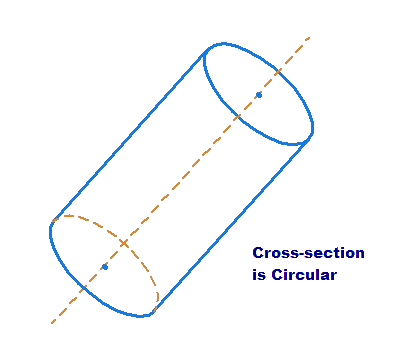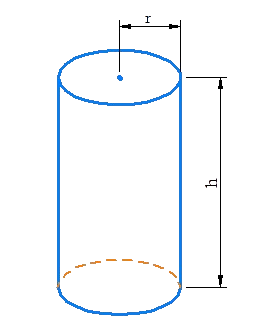# Right Circular Cylinder Basics

Go back to  'Mensuration-Solids'

The following figure shows a right circular cylinder:Note that the cross-section of a right circular cylinder is a circle, and the axis of the cylinder is perpendicular to the base.

Below is an example of a right cylinder which is not circular (the cross-section is not a circle):Below is an example of a circular cylinder which is not right (the cross-section is circular, but the axis is not perpendicular to the base):From now on, we will only discuss right circular cylinders, and refer to them as simply cylinders. Observe that a cylinder’s dimensions can be specified by two parameters: the radius r of the flat face (or the base), and its height h, as shown below.## What is a right circular cylinder?

• A right circular cylinder is a cylinder whose base is a circle and whose elements are perpendicular to its base. Properties of a Right Circular Cylinder. The axis of a right circular cylinder is the line joining the centers of the bases.

## What are the properties of a right circular cyliner?

• A cylinder has 3 faces - 2 circle ones and a rectangle (if you take the top and bottom off a tin can then cut the cylinder part on the seam and flatten it out you would get a rectangle). It has 2 edges and no vertices (no corners)

## What is the Total Surface Area of a hollow cylinder?

• The total surface area of a hollow cylinder is 2π ( r1 + r2 )( r2 - r1 +h), where, r1 is inner radius, r2 is outer radius and h is height.

## What is the difference between a cylinder and a right circular cylinder?

• Cylinder: A prism shaped solid whose bases are circles. Right circular cylinder: A solid shape formed by the segment joining the centers of the circles of a cylinder, which is perpendicular to the planes of the bases.

## What is the total surface area of a right circular cylinder?

• The general formula for the total surface area of a cylinder is T.S.A.=2πrh+2πr^2 .
Mensuration and Solids
Mensuration and Solids
grade 10 | Questions Set 2
Mensuration and Solids
Mensuration and Solids
grade 10 | Questions Set 1